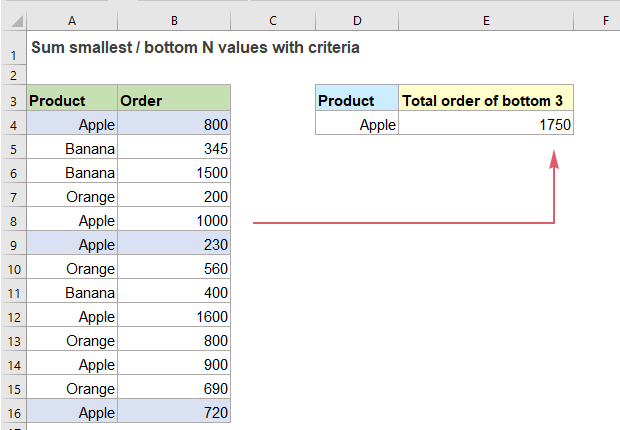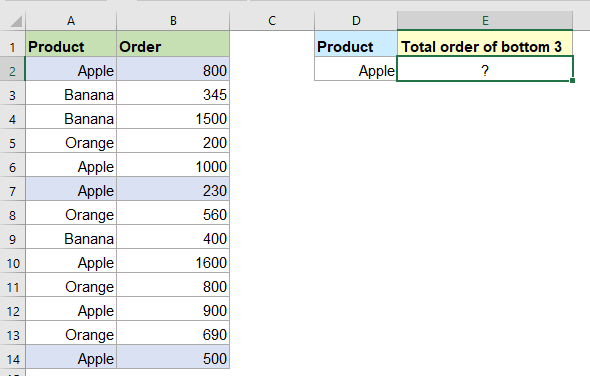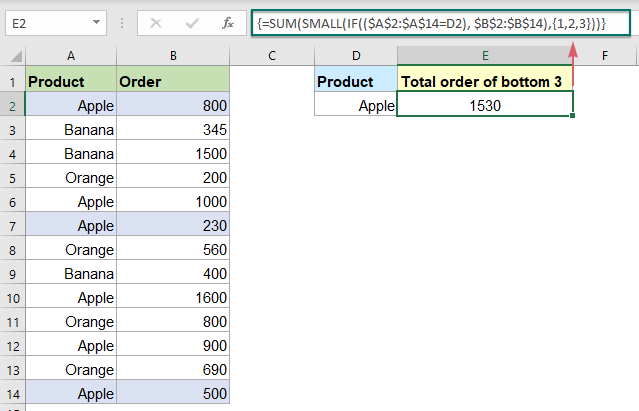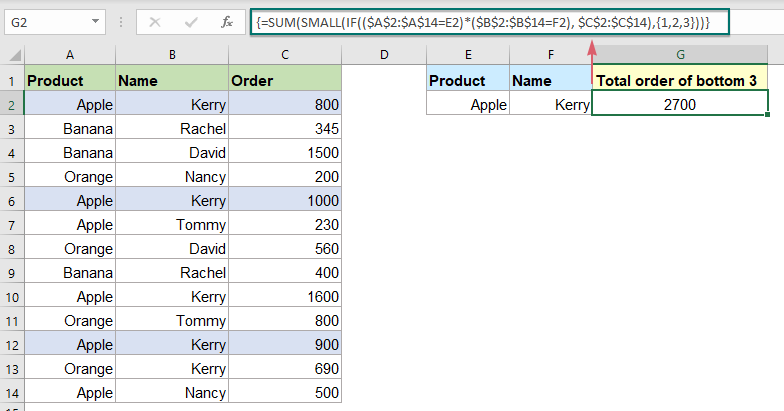## Sum smallest or bottom N values based on criteria in Excel

In previous tutorial, we have discussed how to sum the smallest n values in a data range. In this article, we will perform a further advanced operation – to sum the lowest n values based on one or more criteria in Excel.#### Sum smallest or bottom N values based on criteria in Excel

Supposing, I have a range of data as below screenshot shown, now, I want to sum the lowest 3 orders of the product Apple.In Excel, to sum the bottom n values in a range with criteria, you can create an array formula by using the SUM, SMALL and IF functions. The generic syntax is:

{=SUM(SMALL(IF(range=criteria,values),{1,2,N}))}
Array formula, should press Ctrl + Shift + Enter keys together.
• range=criteria: The range of cells to match the specific criteria;
• values: The list that contains the bottom n values you want to sum;
• N: The Nth bottom value.

To solve the above problem, please apply the below array formula into a blank cell:

=SUM(SMALL(IF((\$A\$2:\$A\$14=D2), \$B\$2:\$B\$14),{1,2,3}))

And then press Ctrl + Shift + Enter keys together to get the correct result as below screenshot shown:##### Explanation of the formula:

=SUM(SMALL(IF((\$A\$2:\$A\$14=D2), \$B\$2:\$B\$14),{1,2,3}))

• IF((\$A\$2:\$A\$14=D2), \$B\$2:\$B\$14): If the product in range A2:A14 equals “Apple”, it will return the relative number from the order list (B2:B14); If the product is not “Apple”, a FALSE will be displayed. You will get the result like this: {800;FALSE;FALSE;FALSE;1000;230;FALSE;FALSE;1600;FALSE;900;FALSE;500}.
• SMALL(IF((\$A\$2:\$A\$14=D2), \$B\$2:\$B\$14),{1,2,3}): This SMALL function will ignore the FALSE values and return the bottom 3 values in the array, so, the result is this: {230,500,800}.
• SUM(SMALL(IF((\$A\$2:\$A\$14=D2), \$B\$2:\$B\$14),{1,2,3}))=SUM({230,500,800}): Finally, the SUM function sums the numbers in the array to get the result: 1530.

Tips: Deal with two or more conditions:

If you need to sum the bottom n values based on two or more criteria, you just need to add other range and criteria by * character within the IF function like this:

{=SUM(SMALL(IF((range1=criteria1)*(range2=criteria2) *(range3=criteria3)…,values),{1,2,N}))}
Array formula, should press Ctrl + Shift + Enter keys together.
• Range1=criteria1: The first range of cells to match the first criteria;
• Range2=criteria2: The second range of cells to match the second criteria;
• Range3=criteria3: The third range of cells to match the third criteria;
• values: The list that contains the bottom n values you want to sum;
• N: The Nth bottom value.

For instance, I want to sum the bottom 3 orders of product Apple that is sold by Kerry, please apply the below formula:

=SUM(SMALL(IF((\$A\$2:\$A\$14=E2)*(\$B\$2:\$B\$14=F2), \$C\$2:\$C\$14),{1,2,3}))

And then press Ctrl + Shift + Enter keys together to get the result as you need:#### Relative function used:

• SUM:
• The SUM function adds values. You can add individual values, cell references or ranges or a mix of all three.
• SMALL:
• The Excel SMALL function returns a numeric value based on its position in a list when sorted by value in ascending order.
• IF:
• The IF function tests for a specific condition and returns the corresponding value that you supply for TRUE or FALSE.

#### More articles:

• Sum Smallest Or Bottom N Values
• In Excel, it is easy for us to sum a range of cells by using the SUM function. Sometimes, you may need to sum the smallest or bottom 3, 5 or n numbers in a data range as below screenshot shown. In this case, the SUMPRODUCT together with the SMALL function can help you to solve this problem in Excel.
• Subtotal Invoice Amounts By Age In Excel
• To sum the invoice amounts based on age as below screenshot shown may be a common task in Excel, this tutorial will show how to subtotal invoice amounts by age with a normal SUMIF function.
• Sum All Number Cells Ignoring Errors
• When summing a range of numbers which contain some error values, the normal SUM function will not work correctly. To sum only numbers and skip the error values, the AGGREGATE function or the SUM along with the IFERROR functions can do you a favor.

### The Best Office Productivity Tools

#### Kutools for Excel - Helps You To Stand Out From Crowd

 Popular Features: Find/Highlight/Identify Duplicates  |  Delete Blank Rows  |  Combine Columns or Cells without Losing Data  |  Round without Formula ... Super VLookup: Multiple Criteria  |  Multiple Value   |  Across Multi-Sheets  |  Fuzzy Lookup... Adv. Drop-down List: Easy Drop Down List  |  Dependent Drop Down List  |  Multi-select Drop Down List... Column Manager: Add a Specific Number of Columns  |  Move Columns  |  Unhide Columns  |  Compare Columns to Select Same & Different Cells ... Featured Features: Grid Focus  |  Design View  |  Big Formula Bar  |  Workbook & Sheet Manager  |  Resource Library (Auto Text)  |  Date Picker  |  Combine Worksheets  |  Encrypt/Decrypt Cells  |  Send Emails by List  |  Super Filter  |  Special Filter (filter bold/italic/strikethrough...) ... Top 15 Toolsets:  12 Text Tools (Add Text, Remove Characters ...)  |  50+ Chart Types (Gantt Chart ...)  |  40+ Practical Formulas (Calculate age based on birthday ...)  |  19 Insertion Tools (Insert QR Code, Insert Picture from Path ...)  |  12 Conversion Tools (Numbers to Words, Currency Conversion ...)  |  7 Merge & Split Tools (Advanced Combine Rows, Split Cells ...)  |  ... and more

Kutools for Excel Boasts Over 300 Features, Ensuring That What You Need is Just A Click Away...

Supports Office/Excel 2007-2021 and 365   |   Available in 44 Languages   |   Easy to Uninstall Completely#### Office Tab - Enable Tabbed Reading and Editing in Microsoft Office (include Excel)

• One second to switch between dozens of open documents!
• Reduce hundreds of mouse clicks for you every day, say goodbye to mouse hand.
• Increases your productivity by 50% when viewing and editing multiple documents.
• Brings Efficient Tabs to Office (include Excel), Just Like Chrome, Edge and Firefox.